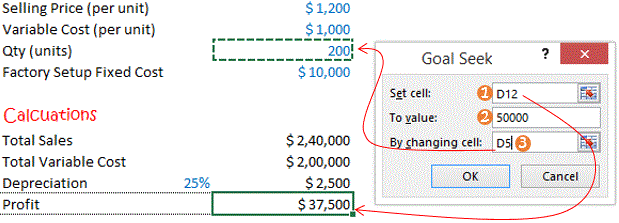Goal seek is one of the incredibly simple (& powerful) features in excel. Let’s try that out today! When you use Goal seek all you are supposed to know is what result do you want and depending on that result you can change input variables… too much talk huh? Let’s pick up a case and get it sorted!

### Here is a quick caseAbove is some information about a factory. It is pretty straight forward (I guess).. doesn’t need too much of explanation. With this information let’s calculate the following1. Total Sales = Qty x Selling Price (per unit)
2. Total Variable Cost = Qty x Variable Cost (per unit)
3. Depreciation = 25%(assumed) x Fixed Cost
4. Profit = Total Sales(1) Total Variable Cost(2) Depreciation(3)

### How does goal seek come into picture

What if I asked you the following questions

1. Can you tell me how much I need to sell (in units), if I wish to make a profit of \$50,000?
2. Or how much should be my (per unit) variable cost, if my profit is only \$25,000?
3. Or to earn a profit of \$40,000 what should be the per unit pricing?

In all the above questions, you know what profit do you want and based on that you want to know how the variables like Qty or Selling price or Variable Price would change.. Goal seek comes in here! (You have the Goal and you are seeking how the variables would change!)

### Applying Goal Seek

Where is Goal seek – In the  Data Tab, under What If Analysis lies Goal Seek Option. Click on Goal SeekWhen you click on Goal Seek the goal seek window appearsLets see what these options (1,2,3) mean and how can you play with them in different scenarios

1. Set Cell: This is always always a formula driven value (in our case- calculated profit). Why? because that’s how you tell excel what formula/calculation method you have used so that excel can use the same to back calculate as for your desired analysis
2. To Value: Here you have to input what new value would you like to give for the cell linked in the set cell field (which is profit in this case). In our case we would like set the new profit to \$ 50,000. Remember this has to be punched manually
3. By Changing Cell: You can link which ever variable you want to change. This could be Qty, Selling price per unit or the Variable cost per unit. The variable (cell) that you select should not be be driven by a formula
4. As soon as you press Enter/Click on OK it would revise the Profit and Qty values

### Goal Seek Output• The Profit has now been changed to \$50,000
• And the Qty (units) have now changed to 262.5 from 200 (earlier)
• If you Click on OK it would replace the old values with the new values